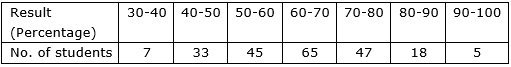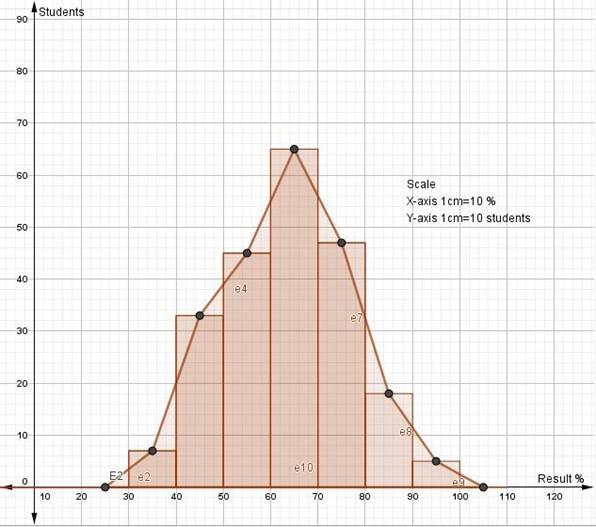SSC BOARD PAPERS IMPORTANT TOPICS COVERED FOR BOARD EXAM 2024

### Practice Set 6.5 Statistics Class 10th Mathematics Part 1 MHB Solution

Practice Set 6.5

Observe the following frequency polygon and write the answers of the questions below…

Show the following data by a frequency polygon.

The following table shows the classification of percentages of marks of students and…

###### Practice Set 6.5

Question 1.

Observe the following frequency polygon and write the answers of the questions below it.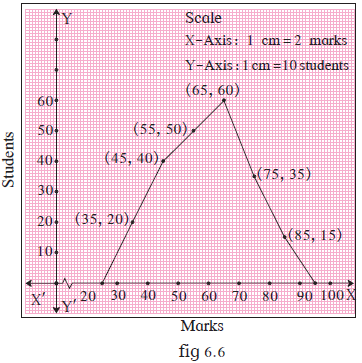(1) Which class has the maximum number of students?

(2) Write the classes having zero frequency.

(3) What is the class-mark of the class, having frequency of 50 students?

(4) Write the lower and upper class limits of the class whose class mark is 85.

(5) How many students are in the class 80-90?

(1) Class 60-70 has maximum number of students

(2) Class 20-30 and class 90-100 have zero frequency

(3) Frequency 50 students is for class 50-60

Class mark for this class is 55

(4) Class mark is 85 for class 80-90

Lower limit = 80

Upper limit = 90

(5) There are 15 students in class 80-90

Question 2.

Show the following data by a frequency polygon.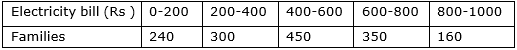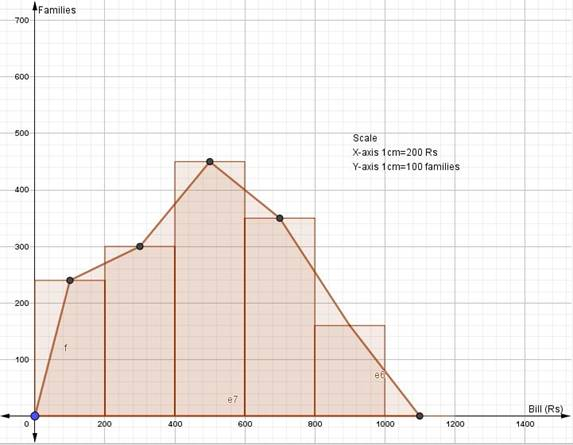Question 3.

The following table shows the classification of percentages of marks of students and the number of students. Draw a frequency polygon from the table.